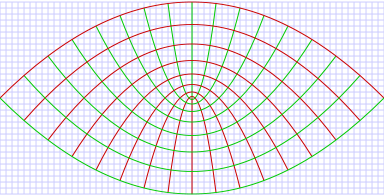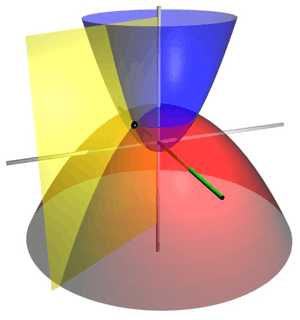# Parabolic coordinatesParabolic coordinates are a two-dimensional orthogonal coordinate system in which the coordinate lines are confocal parabolas. A three-dimensional version of parabolic coordinates is obtained by rotating the two-dimensional system about the symmetry axis of the parabolas.

Parabolic coordinates have found many applications, e.g., the treatment of the Stark effect and the potential theory of the edges.

## Two-dimensional parabolic coordinates

Two-dimensional parabolic coordinatesare defined by the equations, in terms of cartesian coordinates:The curves of constantform confocal parabolaethat open upwards (i.e., towards), whereas the curves of constantform confocal parabolaethat open downwards (i.e., towards). The foci of all these parabolae are located at the origin.

## Two-dimensional scale factors

The scale factors for the parabolic coordinatesare equalHence, the infinitesimal element of area isand the Laplacian equalsOther differential operators such asandcan be expressed in the coordinatesby substituting the scale factors into the general formulae found in orthogonal coordinates.

## Three-dimensional parabolic coordinatesCoordinate surfaces of the three-dimensional parabolic coordinates. The red paraboloid corresponds to τ=2, the blue paraboloid corresponds to σ=1, and the yellow half-plane corresponds to φ=-60°. The three surfaces intersect at the point P (shown as a black sphere) with Cartesian coordinates roughly (1.0, -1.732, 1.5).

The two-dimensional parabolic coordinates form the basis for two sets of three-dimensional orthogonal coordinates. The parabolic cylindrical coordinates are produced by projecting in the-direction. Rotation about the symmetry axis of the parabolae produces a set of confocal paraboloids, the coordinate system of tridimensional parabolic coordinates. Expressed in terms of cartesian coordinates:where the parabolae are now aligned with the-axis, about which the rotation was carried out. Hence, the azimuthal angleis definedThe surfaces of constantform confocal paraboloidsthat open upwards (i.e., towards) whereas the surfaces of constantform confocal paraboloidsthat open downwards (i.e., towards). The foci of all these paraboloids are located at the origin.

The Riemannian metric tensor associated with this coordinate system is## Three-dimensional scale factors

The three dimensional scale factors are:It is seen that The scale factorsandare the same as in the two-dimensional case. The infinitesimal volume element is thenand the Laplacian is given byOther differential operators such asandcan be expressed in the coordinatesby substituting the scale factors into the general formulae found in orthogonal coordinates.